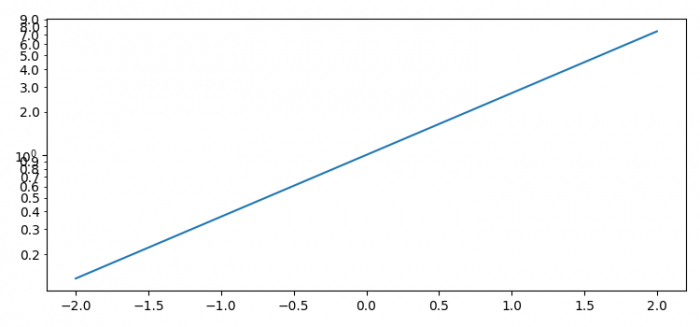# How to show minor tick labels on a log-scale with Matplotlib?

To show minor tick labels on a log-scale with Matplotlib, we can take the following steps −

• Set the figure size and adjust the padding between and around the subplots.
• Create x and y data points using numpy.
• Plot x and y data points using plot() method
• Get the current axis using gca() method.
• Set the yscale with log class by name.
• Change the appearance of ticks and tick label using ick_params() method.
• Set the minor axis formatter with format strings to format the tick.
• To display the figure, use show() method.

## Example

import numpy as np
import matplotlib.pyplot as plt
from matplotlib.ticker import FormatStrFormatter

plt.rcParams["figure.figsize"] = [7.50, 3.50]
plt.rcParams["figure.autolayout"] = True

x = np.linspace(-2, 2, 10)
y = np.exp(x)

plt.plot(x, y)

ax = plt.gca()
ax.set_yscale('log')

plt.tick_params(axis='y', which='minor')
ax.yaxis.set_minor_formatter(FormatStrFormatter("%.1f"))

plt.show()

## Output